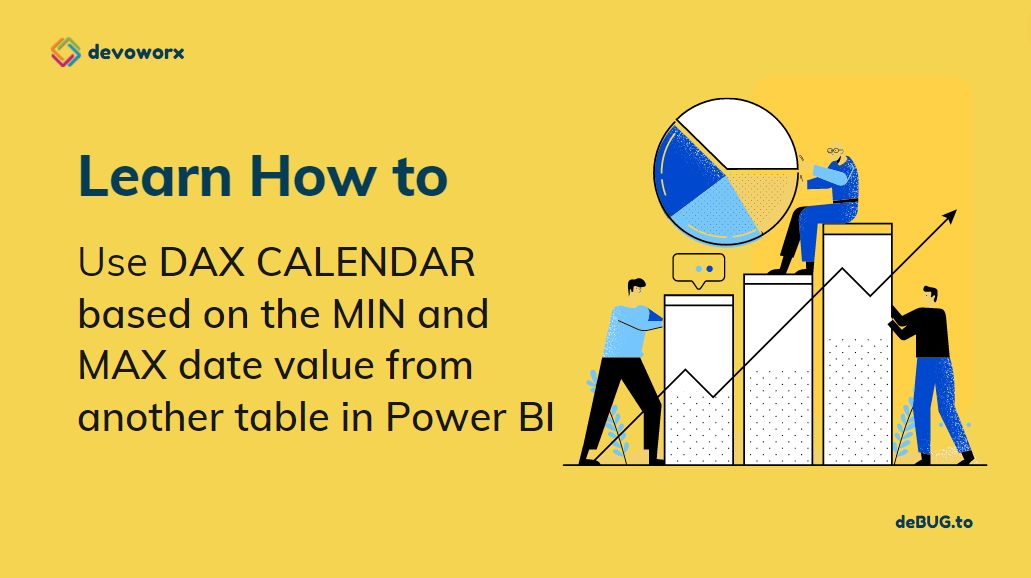## How to use DAX Calendar function in Power BI?

In this article, you will learn how to create an auto-generated date table using the DAX CALENDAR function in Power BI based on a date range or based on the MIN and MAX date value from another table.

## What is DAX in Power BI?

Data Analysis Expressions (DAX) is a formula expression language explicitly designed for the management of data models, the DAX formulas include functions and operators that can be used to create formulas and expressions in Analysis Services, Power BI, and Power Pivot in Excel.

DAX in Power BI includes a library of over 200 functions, operators, that are used by report designers to perform data analysis and calculations.

## What is CALENDAR function in Power BI?

Power BI CALENDAR function is one of the date and time functions in DAX that are similar to date and time functions in Excel.

Using the CALENDAR () function in DAX is one of the simplest ways to create a calendar table as it is a straightforward function with only two input parameters: START and END dates and the date range extend from the specified start date to the specified end date.

You might also like to read How to use Power BI DAX CALENDERAUTO?

### Syntax

``CALENDAR(<start_date>, <end_date>) ``

The start_date must be less than end_date, otherwise it will return error if start_date is greater than end_date

### Output

The CALENDAR function in Power BI will return a single table with one column of dates that extends from the specified start date to the specified end date with one day at each row.

### Power BI DAX CALENDAR function Examples

In this section, we will provide two examples to learn how to use the CALENDAR DAX function in Power BI:

1. Using Power BI DAX CALENDAR function to auto-generate a CALENDAR table based on range.
2. Using Power BI DAX CALENDAR function to auto-generate a CALENDAR table based on another column.

#### Example1:

In this example, we will use the DAX Calendar function to return a table of dates from the start date 1/12022 to the end data 1/19/2022 as below:

##### Steps
1. In Power BI Desktop, Click on Data Mode.
1. In “Table Tools” tab, click on “New Table” to create a new Table using DAX.
1. In the formula box, at the below formula with your date value range as you prefer
``CALENDAR = CALENDAR(DATE(2022,1,1), DATE(2022,1,19))``

Note, you have to provide the date value format is year, month, day

1. As you can see, an auto-generated table has been created based on the specified date range.

#### Example2:

Consider you have a table that holds a date column, and you would like to create a CALENDAR table based on the Minimum and Maximum date range on this column!

In this example, you can also use the CALENDAR function based on a column, and the start date is the minimum date in that column and the end date is the maximum date.

##### Steps
1. In Power BI Desktop, Click on Data Mode.
1. In “Table Tools” tab, click on “New Table” to create a new Table using DAX.
1. In the formula box, at the below formula with your date value range as you prefer
``CALENDAR Table = CALENDAR(FIRSTDATE(sales[Sales Date].[Date]),LASTDATE(sales[Sales Date].[Date]))``
1. As you can see a new CALENDAR table has been created based on the Maximum and Minimum date range in the “Sales Date” column.

#### Conclusion

In conclusion, we have learned How to use DAX Calendar function in Power BI. also, we have provided two examples to explain how to use DAX Calendar function in Power BI.

• Auto-generate a CALENDAR table based on range.
• Auto-generate a CALENDAR table based on another column.